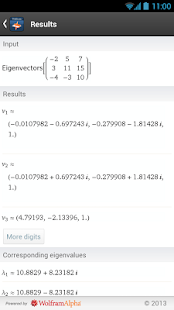Studying linear algebra? Then you need the Wolfram Linear Algebra Course Assistant. This definitive app for linear algebra—from the world leader in math software–will help you work through your homework problems, ace your tests, and learn linear algebra concepts. Forget canned examples! The Wolfram Linear Algebra Course Assistant solves your specific algebra problems on the fly.

This app covers the following topics applicable to linear algebra:
– Solve an equation or a system of equations
– Add or subtract any two vectors
– Find the cross product or dot product for two vectors
– Find the dimensions, transpose, adjugate, rank, inverse, determinant, and reduced row echelon form of a matrix
– Calculate a matrix product
– Compute linear transformations
– Determine subspaces, including row space, column space, and null space
– Find the characteristic equation, eigenvalues, and eigenvectors of a matrix

The Wolfram Linear Algebra Course Assistant is powered by the Wolfram|Alpha computational knowledge engine and is created by Wolfram Research, makers of Mathematica—the world’s leading software system for mathematical research and education.

The Wolfram Linear Algebra Course Assistant draws on the computational power of Wolfram|Alpha’s supercomputers over a 2G, 3G, 4G, or Wi-Fi connection.

What’s New

• Bug fixes

Screenshots

•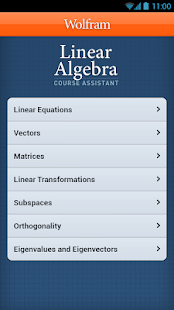•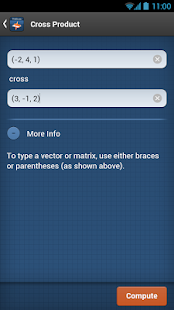•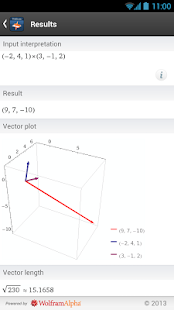•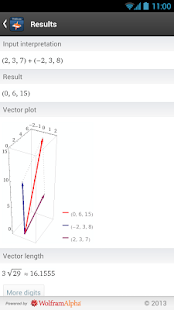•